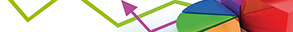# Modeling and Applications of Regularized Regression

Publishing date
01 Sep 2020
Status
Open
08 May 2020

Guest Editors

3IIT Kanpur, Kanpur, India

# Modeling and Applications of Regularized Regression

## DescriptionThe identification of significant independent variables plays a crucial role in building a multiple regression model for many real-world applications. This variable selection process allows the model to perform better in multiple directions including better prediction of the dependent variable and correctly portraying the role of independent variables which are important to fitting a model. However, classical variable selection methods, such as Akaike information criterion (AIC), Mallow’s Cp, and Bayesian information criterion (BIC), are often highly time-consuming and suffer from potential instability.

Recently, Markov Chain Monte Carlo- (MCMC-) based computation techniques for subset selection, using stochastic search variable selection (SSVS) algorithms, have become widely used in linear regression, generalized linear models, Quality Requirement (QR) models, and other modeling frameworks. However, for some applications, such as chemometrics or bioinformatics, SSVS algorithms are very time-consuming, and consequently this method suffers from computational difficulties. Therefore, variable selection by regularization methods has received substantial interest in recent years.

The aim of this Special Issue is to focus on regularized regression methods, specifically on the role of the regularization in variable selection and estimation in linear regression models. In addition, submissions on asymptotic theories with diverging number of parameters are encouraged. Both original research articles highlighting advances in the modeling and applications of regularized regression and review articles on the current state of the art are welcome.

Potential topics include but are not limited to the following:

• Regularized least squares regression
• Regularized robust regression methods
• Regularized quantile regression methods
• Regularized expectile regression
• Bayesian regularized regression
• Bayesian regularized quantile regression
• Bayesian regularized expectile regression
• Optimal rates for regularized least squares regression
• Asymptotic oracle properties of the regularized estimators
• Overcoming the overfitting problems using the regularization methodsJournal metrics
Acceptance rate7%
Submission to final decision44 days
Acceptance to publication89 days
CiteScore0.560
Impact Factor-